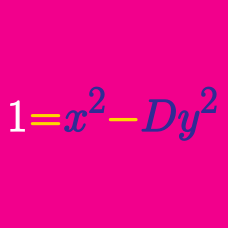Number Theory

# Quadratic Diophantine Equations: Level 2 Challenges

Magician Mike Michael claims to know two positive whole numbers that multiply to 1000, neither of which contain the digit 0.

What is the sum of these 2 numbers?

$\large a!b! = a! + b!$

If $a$ and $b$ are positive integers that satisfy the equation above, find $a + b$.

If we mistakenly add numerator and denominators, we would think:

$\Large \dfrac{1}{a} + \dfrac{1}{b}=\dfrac{2}{a+b}.$

How many ordered pairs $(a.b)$ in the interval $-10\leq a,b \leq 10$ such that they satisfy the equation above.

What is the smallest positive multiple of hundred which can be expressed as the product of two consecutive integers?

How many primes are 4 less than a perfect square?

×